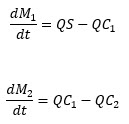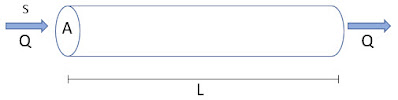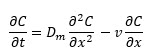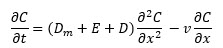## November 13, 2020

### GoldSim Contaminant Transport Module Online Course, Excerpt #4: Physical Processes Controlling Mass Transport

Posted by Rick Kossik

As I noted in previous blog posts, I am currently working on an online CT Course. In order to provide some useful material for users in the meantime, I plan to post excerpts from time to time as I slowly progress through the development of the Course.

The first excerpt simply provided the outline describing how the Course will be organized.  The second excerpt  discussed the key decisions required before starting to build a contaminant transport model. The third excerpt discussed the problem of uncertainty in contaminant transport models and the underlying philosophy of the GoldSim Contaminant Transport Module.

This excerpt finally starts to get a bit more technical, and provides an overview of the physical processes controlling mass transport (advection, dispersion and diffusion).

The cornerstone of any mass transport model is the concept of mass balance or mass conservation. When mass transport equations are solved, it is necessary to discretize space into discrete volumes or compartments. Mass is spread out equally throughout any particular discretized volume instantaneously (i.e., the volume is instantaneously well-mixed). For each such compartment in the model (and for each chemical or contaminant being considered) the mass balance equation takes the following form:

(Rate of change of mass) = (mass transport in) – (mass transport out) + (mass production internally) – (mass elimination internally)

To discuss the various processes here, we will consider this equation for some very simple examples.

Let’s first consider two tanks, each with a constant volume of V:The tanks are always completely and instantaneously mixed and water flows through the tanks at a constant volumetric rate of Q (such that the volumes remain constant).  Initially, both tanks have no chemical mass in them, but at some point we start to inject mass into the inflow to Tank 1 (such that the inflow has a constant concentration of S). The system includes only the two tanks (and the pipe connecting them). There is a boundary upstream of Tank 1 (the mass flowing in is an external forcing function) and there is a boundary downstream of Tank 2 (we no longer track the mass after it leaves Tank 2).

Given this information, we can write the mass balance equations (one for each compartment, in this case, each tank) in the form of differential equations to represent this system.  We will write these equations in terms of the mass of chemical, M, and the concentration, C, in each of the two tanks:We can use the volume to convert concentrations to mass, and rewrite these equations in terms of only the mass:Thinking back to your calculus class, you may recognize these as a system of coupled first-order linear ordinary differential equations.

The QC (or QM/V) terms represent advection. Advection is the transport of material (e.g., contaminants) via the bulk movement of the medium (typically water) in which those materials are dissolved or suspended. Advection will typically be the dominant transport processes in systems that you will model.

In the simple example described above, we had two equations, one for each “location”, in this case two tanks, each of which were assumed to be well-mixed.  However, what if we had a physical situation in which we could not assume such a well-mixed system?  For example, imagine a pipe of cross-sectional area A and length L in which water enters at one end and exits at the other end:In this case, if we introduced mass into the pipe at the upstream end at a constant concentration S (uniformly across the entire cross-sectional area), the concentration would subsequently vary along the pipe from 0 to L.  Let’s assume that we can treat the system as fully one-dimensional (i.e., the flow rate and the concentration is constant across a cross-sectional plane normal to the pipe). In this case, it can be shown that we could write the governing equation describing that concentration as follows:v is the velocity of the flowing water (equal in this simple example to Q/A, where Q is the flow rate and A is the cross-sectional area), and x is the distance along the pipe (from 0 to L).

(If you are interested, a nice explanation of the derivation of this - as well as the other equations we will discuss below - is provided in Applied Contaminant Transport Modeling by Zhen and Bennett.)

In this equation, C represents the concentration at any point along the pipe (defined by x). Note that this is a partial differential equation, in that C is a function of both time and distance along the pipe. (To fully describe this system, we would also need to specify boundary conditions at the upstream and downstream ends of the pipe, but for the purposes of the present discussion, we don’t need to worry about this.)

The key point to note is that this is a very different type of equation than when we could simply assume two well-mixed separate tanks.  In particular, in this case, the concentration varies continuously over the pipe, and the governing equation (the advective mass transport rate) is defined in terms of a concentration gradient (and if we did not impose the assumptions that allowed us to treat the system in only one dimension, we would need to consider concentration gradients in all three dimensions). That is, whereas there was an implicit spatial component when we considered the two tanks (since the two tanks are in fact spatially separate), in this case there is now an explicit spatial component to the equation. This will require a different approach to solving the equation than in the tank example.

One final comment about this equation: in the real world this equation would be unlikely to accurately describe the actual concentration variation in time and distance along the pipe. If this truly was the correct description of the system, assuming the pipe started with no contaminant present, and we started adding contaminant at a constant rate at the upstream end, if we monitored the end of the pipe, we would see that the concentration leaving the pipe would go from 0 to S (where S is the concentration added at the upstream end) in a sharp vertical step (as shown in the red curve below). This plot of concentration versus time at the end of the pipe is referred to as a breakthrough curve.In the real world, however, we would see that this breakthrough would be spread out or dispersed to some extent (as shown in the blue curve). That is, realistically, we can’t explain the behavior using advection alone and need to also represent whatever process is causing this dispersion.

# Diffusion and Dispersion

We noted above that when a chemical is transported through a pipe as in our simple example, we would not be able to explain its breakthrough curve only using advection. We noted that the breakthrough curve would be dispersed. This dispersion is due to the fact that not all of the molecules of the chemical would move longitudinally along the pipe at the same rate.

In order to understand how we describe and quantify this, let’s simplify our pipe example even further. In particular, let’s assume we inject a slug of mass (uniformly over the entire cross-sectional area) at one end of the pipe, but do not allow any water to flow through the pipe at all. That is, both ends of the pipe are closed and there is no advection. What would happen to the concentration of the chemical in the pipe?

What would happen, of course, is that the molecules would diffuse across the pipe (due to the random thermal motion of the molecules) until eventually the entire pipe was at a uniform concentration.  These random movements can be described statistically, and the evolution of the concentration in the pipe can be described using Fick’s Second Law:Dm is the molecular diffusion coefficient (dimensions of L2/T). Molecular diffusion through water is a very slow process (diffusion of the length of a meter would take on the order of 10 years). Nevertheless, as we will discuss in later Units, this process can be critical for some applications, particularly those where engineered diffusive barriers are part of the design and the models have time scales of interest that are very large (e.g., radioactive waste management applications).

So let’s return to our pipe example again, and now consider both advection and diffusion. If we combine the diffusive and advective terms, our equation for concentration in the pipe looks like this:Even though we have now included an additional term in our equation, due to the fact that diffusion is such a slow process, we would find that our simulated dispersion would still be quite low (the breakthrough curve if we solved this equation would be still very close to a vertical line).  The dimensionless Peclet number can be used to compare the importance of advection to diffusion:where v is the advective velocity, L is the distance of interest and Dm is the diffusion coefficient. Hence, over a distance of a meter, and assuming a diffusion coefficient of 1E-9 m2/sec (a typical value for most chemicals), the advective velocity would need to be 1E-9 m/sec in order for advection and diffusion to be comparable!  This indicates that diffusion is generally only of interest in the absence of advection. (Note, however, that there is one special type of diffusive transport process - matrix diffusion, which is diffusion perpendicular to the direction of advective flow - that is important for some types of systems.  In this special case, diffusion can indeed have a substantial impact on advective systems.)

So if diffusion does not cause the dispersion we said we would see in an actual pipe, what does cause it?  There are actually two other processes that can cause dispersion (and these, when present, are generally many orders of magnitude greater than molecular diffusion).  Mathematically these are treated in the same manner as molecular diffusion. These processes are turbulent (or eddy) diffusion and mechanical dispersion.

Turbulent water (or air) motions consist of constantly changing swirls of fluid of different sizes, referred to as eddies. These are small-scale fluctuations superimposed on the large-scale movement of the fluid. Although we cannot describe these movements exactly, they can be described statistically in the same way that molecular diffusion can be described statistically. That is, turbulent diffusion has the effect of carrying mass in the decreasing direction of concentration (in exactly the same manner as molecular diffusion does).

For flow through porous media, the fluid is generally moving much more slowly and is not turbulent. But the groundwater must take detours around the various solid particles, and these detours result in mixing, referred to as mechanical dispersion. This kind of dispersion can also take place at a larger scale than that of the individual particles, as water may flow around large-scale regions of less permeable material (referred to as macrodisperson).  Like molecular diffusion and turbulent diffusion, mechanical dispersion has the effect of carrying mass in the decreasing direction of concentration.

The total effective Fickian transport coefficient is the sum of these three processes:where Dm is the molecular diffusion coefficient, E is the eddy diffusion coefficient and D is the mechanical dispersion coefficient (and all three have dimensions of L2/T). Typically, one of these dominates for any particular situation.

The molecular diffusion coefficient is a function of the fluid, the size of the chemical diffusing and the temperature.  As we shall see in a subsequent Unit, when diffusing through a porous medium, the properties of the porous medium (e.g., porosity, tortuosity, saturation) also impact the value. Eddy diffusion coefficients and mechanical dispersion coefficients depend not on the properties of the chemical, but on site-specific flow conditions (and hence can vary with time and location and be anisotropic). They also tend to be scale-dependent (i.e., the values grow as the size of the system increases due to processes such as macrodispersion mentioned above).

Focusing on transport through porous media, the mechanical dispersion coefficient, D, is often approximated as:where α is the (longitudinal) dispersivity (dimensions of L) and v is the seepage velocity (dimension of L/T), defined as Q/(θA), where Q is the volumetric flow rate, A is the cross-sectional area perpendicular to flow and θ is the porosity of the porous medium. As noted above, the dispersivity is often treated as scale-dependent.

As will be discussed in great detail in subsequent Units, GoldSim can easily represent all of these physical transport processes (advection, dispersion and diffusion).